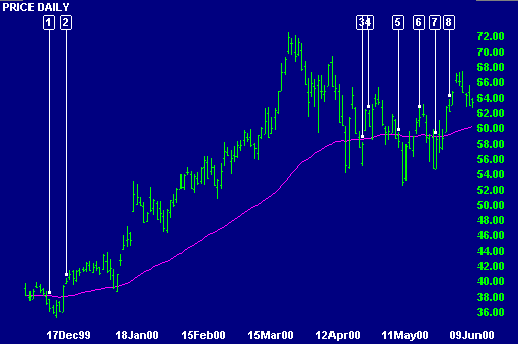# Moving Average Filters

Moving averages are prone to whipsaws, when price crosses back and forth across the moving average in a ranging market. Traders have developed a number of filters over the years to eliminate false signals.

The simplest moving average system generates signals when price crosses the moving average:

• Go long when price crosses to above the moving average from below.
• Go short when price crosses to below the moving average from above.

### Filters

Filters are added to objectively measure when price has crossed the moving average. The most common filters are:

### Moving Average Slope

Trades are only entered if the moving average slopes in the direction of the trade. This filter will not work with exponential moving averages because the exponential moving average always slopes up when price closes above the moving average and slopes down if it closes below.

Exit when price re-crosses the moving average.

Moving Average Slope can be used in conjunction with other filters such as closing price.

### Example

Intel Corporation is plotted with a   63 day exponential moving average.

The single moving average is used with two filters:Mouse over chart captions to display trading signals.

1. Go short - two closes below a falling moving average.
2. Go long - moving average is now rising and price has closed above the moving average for 2 days.
The following dip below the moving average (in early January) is filtered out.
3. The long trade is exited as there are two closes below the moving average.
No short trade is entered as the moving average is sloping upwards.
4. Go long - two closes above a rising moving average.
5. Go short as there are two closes below a falling moving average.
6. Go long - two closes above a rising moving average.
7. Go short - two closes below a falling moving average.
8. Go long - moving average is rising again and there are 2 closes above it.

Note how profitable the long trade  is during the strong upward trend, compared to when price whipsaws around the relatively flat moving average, frequently switching you in and out of trades. Trend indicators are normally unprofitable, and should be avoided, during ranging markets.